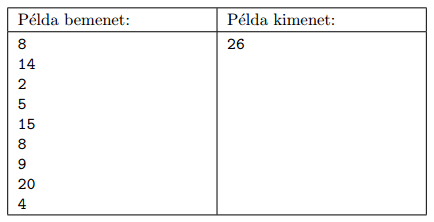Mathematical and Physical Journal
for High Schools
Issued by the MATFUND Foundation
 Already signed up? New to KöMaL?

#Problem S. 91. (September 2014)

S. 91. Alice, Bob and Cecil are given $\displaystyle N$ ($\displaystyle 1\le N\le 60$) boxes of presents. The value of each box is known: the $\displaystyle i$th box is worth $\displaystyle a_i$ with $\displaystyle 1\le a_i\le 100$. They would like to distribute these presents in a fair way. A particular distribution of the presents among the three people is said to be fair, if the person getting the set of presents with the highest total value gets a set of presents having the smallest possible total value. For example, if the presents have values 2, 4, 5, 8, 9, 14, 15 and 20, then a fair distribution could be the following: Alice gets the presents with values 2, 9 and 15 (the total sum is 26); Bob gets the presents 4, 8 and 14 (with total sum 26); and Cecil gets the presents 5 and 20 (with total sum 25). Given the list of presents, your task is to determine the maximal sum of presents a person can get during a fair distribution (in other words, that maximal sum is to be minimized). Your program should read $\displaystyle N$ from the first line of the standard input, then the $\displaystyle a_i$ integers (separated by a space) from the following $\displaystyle N$ lines. The first and only line of the standard output should contain the minimal maximum value in a fair distribution. In the example, Példa bemenet'' is a sample input, while Példa kimenet'' is the corresponding output.Scoring and bounds. You can obtain 1 point for a brief and proper documentation clearly describing your solution. Nine further points can be obtained provided that your program solves any arbitrary valid input within 1 second of running time. Partial points can be obtained if your program yields a solution

$\displaystyle \bullet$ for $\displaystyle a_i\le 40$, or

$\displaystyle \bullet$ for $\displaystyle N\le 10$.

The source code (s91.pas, s91.cpp, ...) without the .exe or any other auxiliary files generated by the compiler but with a short documentation (s91.txt, s91.pdf, ...), also describing which developer environment to use for compiling the source, should be submitted in a compressed file s91.zip.

(10 pont)

Deadline expired on October 10, 2014.

### Statistics:

 22 students sent a solution. 10 points: Erdős Márton, Fuisz Gábor, Molnár-Sáska Zoltán, Németh 123 Balázs, Zalavári Márton. 9 points: Kiss Gergely. 8 points: 2 students. 7 points: 4 students. 6 points: 2 students. 5 points: 1 student. 4 points: 1 student. 3 points: 3 students. 2 points: 2 students. 0 point: 1 student.

Problems in Information Technology of KöMaL, September 2014